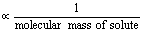1800-1023-196

+91-120-4616500

# Abnormal Molecular Masses

## Abnormal Molecular Masses

Molecular masses can be calculated by measuring any of the colligative properties. The relation between colligative properties and molecular mass of the solute is based on following assumptions.

(1) The solution is dilute, so that Raoult’s law is obeyed.

(2) The solute neither undergoes dissociation nor association in solution.

In case of solutions where above assumptions are not valid we find discrepencies between observed and calculated values of colligative properties. These anomalies are primarily due to

(i)  Association of solute molecules.

(ii)  Dissociation of solute molecules.

(i)  Association of solute molecules: Certain solutes in solution are found to associate. This eventually leads to a decrease in the number of molecular particles in the solutions. Thus, it results in a decrease in the values of colligative properties.

Colligative propertytherefore, higher values are obtained for molecular masses than normal values for unassociated molecules.

(ii) Dissociation of solute molecules : A number of electrolytes dissociate in solution to give two or more particles (ions). Therefore, the number of solute particles, in solutions of such substances, is more than the expected value. Accordingly, such solutions exhibit higher values of colligative properties. Since colligative properties are inversely proportional to molecular masses, therefore, molecular masses of such substances as calculated from colligative properties will be less than their normal values.

## NEET & AIIMS Exam Sample Papers

 AIIMS SAMPLE PAPERS View More NEET SAMPLE PAPERS View More